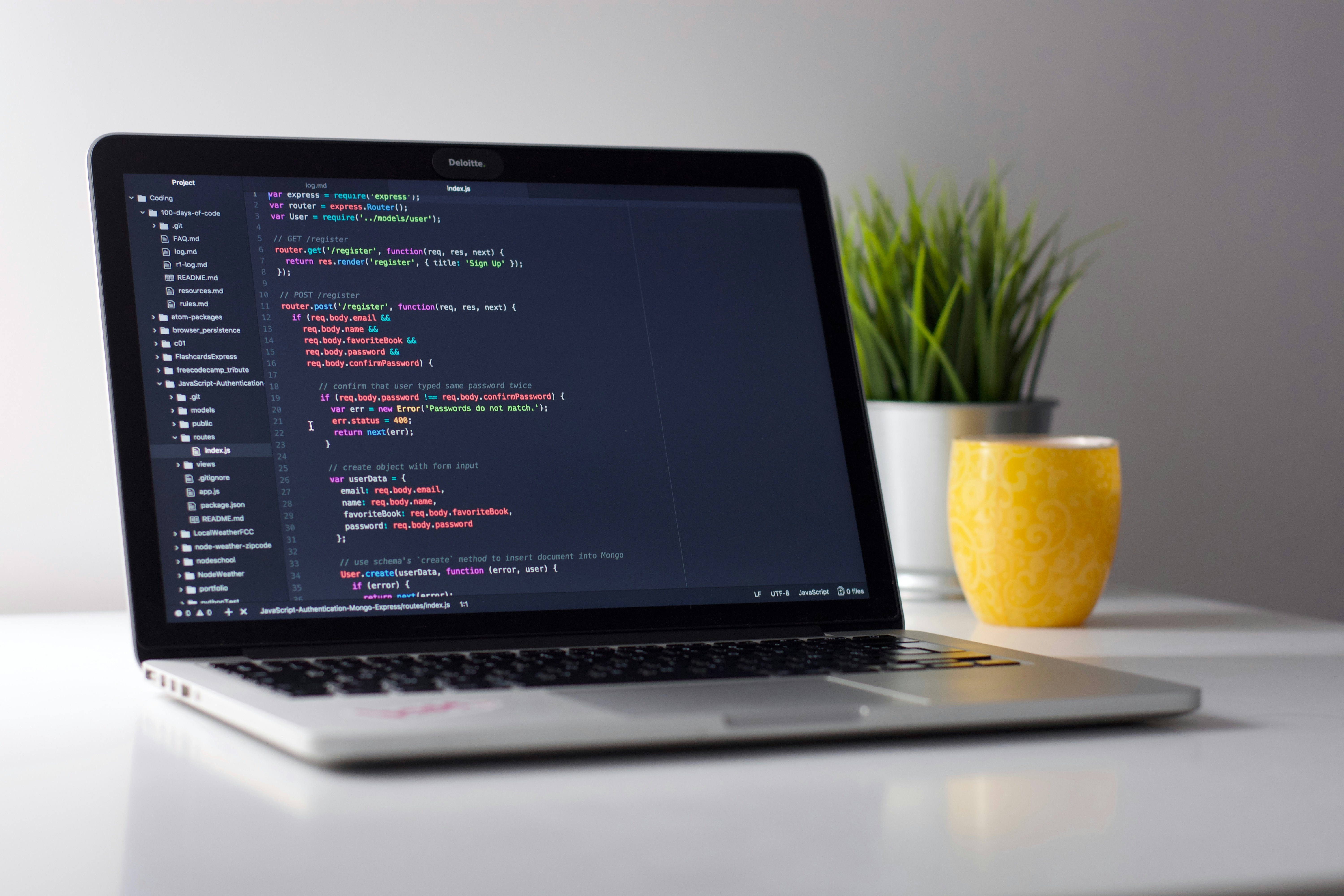Hackerrank Accessing Inherited Functions Solution

# Hackerrank Accessing Inherited Functions SolutionYou are given three classes A, B and C. All three classes implement their own version of func.

In class A, func  multiplies the value passed as a parameter by :

class A
{
public:
A(){
callA = 0;
}
private:
int callA;
void inc(){
callA++;
}

protected:
void func(int & a)
{
a = a * 2;
inc();
}
public:
int getA(){
return callA;
}
};

In class B, func multiplies the value passed as a parameter by :

class B
{
public:
B(){
callB = 0;
}
private:
int callB;
void inc(){
callB++;
}
protected:
void func(int & a)
{
a = a * 3;
inc();
}
public:
int getB(){
return callB;
}
};

In class C, func multiplies the value passed as a parameter by :

class C
{
public:
C(){
callC = 0;
}
private:
int callC;
void inc(){
callC++;
}
protected:
void func(int & a)
{
a = a * 5;
inc();
}
public:
int getC(){
return callC;
}
};

You are given a class D:

class D
{

int val;
public:
//Initially val is 1
D()
{
val = 1;
}

//Implement this function
void update_val(int new_val)
{

}
//For Checking Purpose
void check(int); //Do not delete this line.
};

You need to modify the class D and implement the function update_val  which sets D's val to new_val by manipulating the value by only calling the func defined in classes A, B and C.

It is guaranteed that new_val has only  and  as its prime factors.

Input Format

Implement class D's function update_val. This function should update D's val only by calling A, B and C's func.

Constraints

new_val
Note: The new_val only has  and  as its prime factors.

Sample Input

new_val

Sample Output

A's func will be called once.
B's func will be called once.
C's func will be called once.

Explanation

Initially, val .

A's func is called once:

val = val*2
val = 2


B's func is called once:

val = val*3
val = 6


C's func is called once:

val = val*5
val = 30


### Solution in cpp

Approach 1.

class D:public A,public B,public C
{

int val;
public:
//Initially val is 1
D()
{
val=1;
}

//Implement this function
void update_val(int new_val)
{

int a=new_val;
while ( a %2 == 0)
{
a = a/2;
A::func(val);
}
while ( a % 3 == 0)
{
a = a/3;
B::func(val);
}
while ( a % 5 == 0)
{
a = a/5;
C::func(val);
}
}
//For Checking Purpose
void check(int); //Do not delete this line.
};


Approach 2.

class D : A, B, C
{

int val;
public:
//Initially val is 1
D()
{
val=1;
}

//Implement this function
void update_val(int new_val)
{
while (new_val % 5 == 0) {
new_val /= 5;
C::func(val);
}
while (new_val % 3 == 0) {
new_val /= 3;
B::func(val);
}
while (new_val % 2 == 0) {
new_val /= 2;
A::func(val);
}

}
//For Checking Purpose
void check(int); //Do not delete this line.
};


Approach 3.

class D : public A,public B,public C
{

int val;
public:
//Initially val is 1
D()
{
val=1;
}

//Implement this function
void update_val(int new_val)
{
while(new_val % 2 == 0){
new_val /= 2;
A::func(val);
}
while(new_val % 3 == 0){
new_val /= 3;
B::func(val);
}
while(new_val % 5 == 0){
new_val /= 5;
C::func(val);
}

}
//For Checking Purpose
void check(int); //Do not delete this line.
};